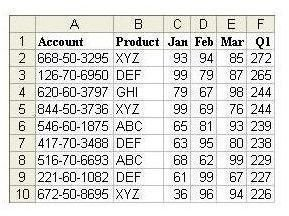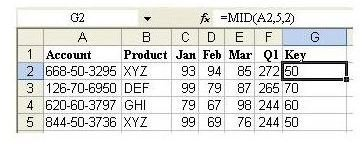# Microsoft Excel: How to Isolate the Middle Portion of a Cell with MID Function

## Using the MID Function to Extract Middle Values

Excel’s MID function is a powerful tool that lets you isolate and extract the contents in the middle of a cell. The following example illustrates how to use this tool by using the MID function to extract the values from the middle of an account number and displaying them in a new column.

Strategy: Insert a new column and use the MID function to isolate the necessary digits from the account field.

The MID function takes three arguments. The first argument is a cell containing a text value. The second argument is the character number where you want the result to start. The final argument is the length of the result.

In a well-formed account number, like 123-45-6789, you can predict that the start of the second segment will always be in the fifth character position. (The "-" counts as a character here.) The length of the second segment is always two characters.

Click any image for a larger view.In a blank column, enter a heading, such as the word “Key” – or anything else that makes sense for your particular table. In cell G2, enter the formula =MID(A2,5,2). Copy the formula down to all rows, as shown in Fig. 267.Additional Details: In order to capture the final four digits of the account number, you could either use the =MID(A2,8,4) or the =RIGHT(A2,4) function to isolate the final four digits of the account number.

Result: You can now sort by the new column and add subtotals by this field.

Summary: When you need to isolate a portion of the characters in another column, creating a temporary column and using the MID function is the solution.

Functions Discussed: =MID(); =RIGHT()

Cross Reference: How to Sort on One Segment of an Account ID

## Additional Resources

For more tips and tricks, be sure to check out the other Microsoft Excel user guides and tutorials found here at Bright Hub. In particular, you may want to browse through the following collections that focus on calculations, functions, formulas, and charts in Excel.

91 Tips for Calculating with Microsoft Excel – This collection of tips is taken straight from the popular book, Learn Excel from Mr. Excel, written by the Excel guru, Bill Jelen. Discover everything from how to use Excel to play games to using Excel to calculate loan payments. You can even use Excel to help your kids with their math homework!

Tips and Tricks for Creating Charts and Graphs in Microsoft Excel – Having trouble formatting a histogram or creating a scatter plot in Excel? Or, do you need to use two chart types in one graphical exhibit? Learn how to do these things and more with this group of detailed chart and graph tutorials.